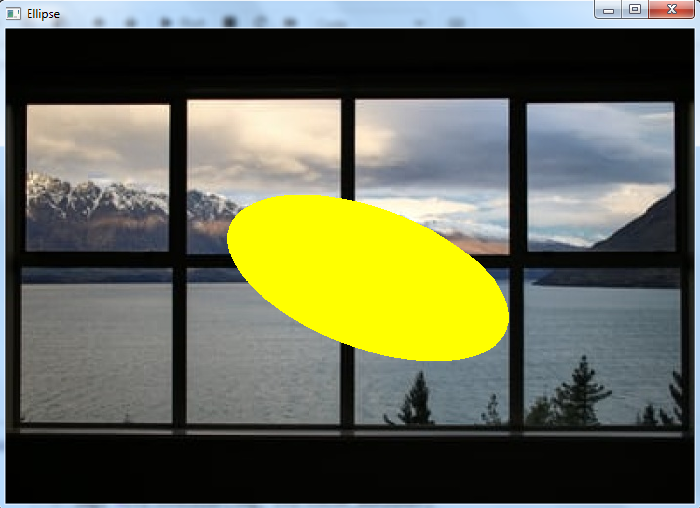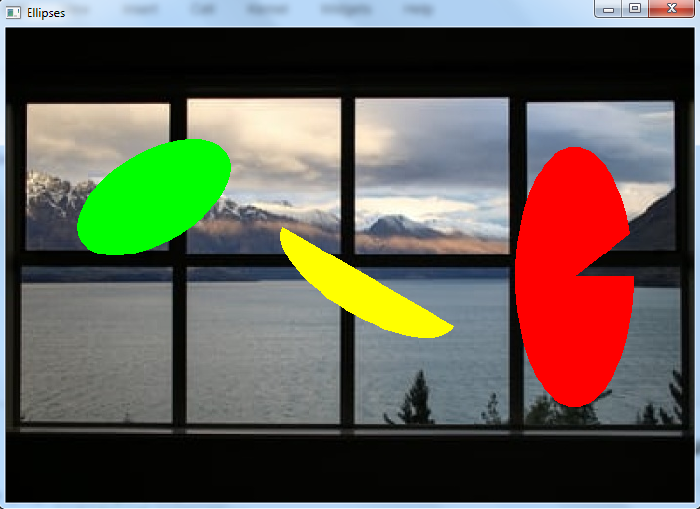# How to draw filled ellipses in OpenCV using Python?

To draw a filled ellipse on an image, we use the cv2.ellipse() method. This method accepts different arguments to draw different types of ellipses.

## Syntax

cv2.ellipse(img,center,axes,angle,start_angle,end_angle,color,thickness)


## Parameters

• img − The input image on which the ellipse is to be drawn.

• center − The center coordinate of the ellipse.

• axes − A tuple in (major axis length, minor axis length) format.

• angle − The rotation angle of an ellipse in degrees.

• start_angle − The starting angle of the elliptic arc in degrees.

• end_angle − The ending angle of the elliptic arc in degrees.

• color − It is the color of the ellipse to be drawn.

• thickness − It is the thickness of the ellipse border line in px. To draw a filled ellipse, set "thickness = -1".

Output − It returns the input image with drawn ellipse/s.

## Steps

You can use the following steps to draw a filled ellipse on the input image −

Import the required library. In all the following Python examples, the required Python library is OpenCV. Make sure you have already installed it.

import cv2


img = cv2.imread('window1.jpg')


Define the parameters to be passed to cv2.ellipse() method. These parameters are img, center, axes, angle, start_angle, end_angle, color, and thickness. To draw a filled ellipse, set "thickness=-1".

cv2.ellipse(img,center,axes,angle,start_angle,end_angle,color,thickness)


Display the image with a drawn ellipse on it.

cv2.imshow("Ellipse", img)
cv2.waitKey(0)
cv2.destroyAllWindows()


Let's look at some examples for clear understanding.

We use the following image as the input file in the examples below.## Example 1

In the Python program below, we draw a filled ellipse on the input image.

# import required libraries
import cv2

# define the arguments
center = (368,250)
axes = (150,70) # major, minor axes
angle = 20
start_angle = 0
end_angle = 360
color = (0,255,255)
thickness = -1

# Draw a filled ellipse on the input image
cv2.ellipse(img,center,axes,angle,start_angle,end_angle,color,thickness)

# display the image with drawn filled ellipse
cv2.imshow("Ellipse", img)
cv2.waitKey(0)
cv2.destroyAllWindows()


## Output

On execution of the above code, it will produce the following output window.The output window shows a filled ellipse drawn on the input image.

## Example 2

In the Python 3 program below, we draw three different filled ellipses on the input image.

import cv2
img1 =cv2.cvtColor(img, cv2.COLOR_BGR2GRAY)
ret,thresh = cv2.threshold(img1,170,255,0)

# draw different shapes of ellipses
img = cv2.ellipse(img,(368,250),(100,40),30,0,180,(0,255,255),-1)
img = cv2.ellipse(img,(150,170),(86,45),-30,0,360,(0,255,0),-1)
img = cv2.ellipse(img,(578,250),(60,130),0,0,340,(0,0,255),-1)

# display the image with drawn ellipses
cv2.imshow("Ellipses", img)
cv2.waitKey(0)
cv2.destroyAllWindows()


## Output

On execution of the above code, it will produce the following output window.The output window shows different types of filled ellipses drawn on the input image. The green ellipse is rotated by "−30°". The yellow ellipse is half drawn and rotated by an angle of 30°. The red ellipse has a minor axis greater than the major axis and ends at 340°.